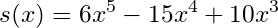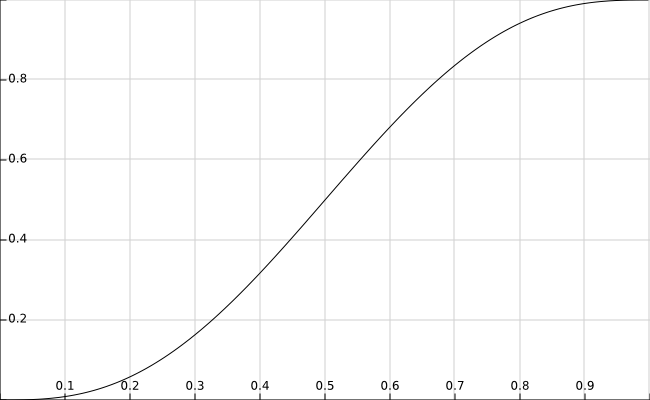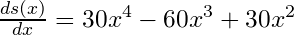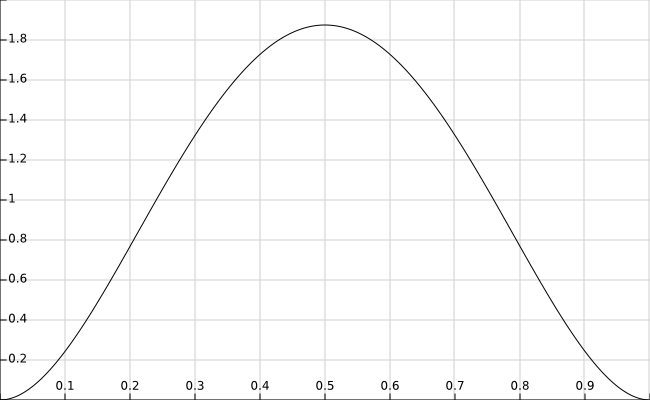Nonsense Placement Mandatory

# npm

## atlas-quintic-smoothing

1.0.0 • Public • Published

# atlas-quintic-smoothing

A commonly used quintic smoothing function for values in the domain [0,1].## install

``````npm install --save atlas-quintic-smoothing
``````

## why

When generating values between an interval, it can be helpful to use a smoothing function to ease values which are close to the endpoints of the interval.

The smoothing function used here is a 5th order polynomial of the form:## examples

#### using smoothing

``````const smooth = require("atlas-quintic-smoothing");

// create a vector of input values
const inputs = [];
for (let x = 0; x <= 1; x+=.001) inputs.push(x);

// apply smoothing
const smoothed = inputs.map(x => smooth(x));
``````

#### output function visualized

Your output values will fall along the following curve:#### understanding smoothing

Smoothing works by changing how the input values change over their interval. For example, `x = .01` and `x = .02` are `delta = .01` units away from each other. When these values are smoothed, they are squeezed together in the output space.

For many smoothing functions (including this one), values in the center of the input range are spread apart. For example `x = .50` and `x = .51` are `delta = .01` units away from each other. However, when they are smoothed, they are almost twice as far away in the output space. Higher order smoothing functions tend to squeeze and stretch values to a greater extent.

A derivative is just a fancy way to say "slope" at some point in our function. First, recall that the derivative of `f(x) = x` is `1`, meaning its slope never changes as a function of `x`. To understand whether values will be squeezed or stretched in the output space, we can take the first derivative of the smoothing function:We want to ask ourselves whether or not the smoothing function will squeeze or stretch our input values at a certain point. All we need to do is plug our point into the derivative above. For example, plugging in the point `x = .1` tells us that the derivative is `s'(.1) = .243`, meaning it grows roughly four times slower than `f(x) = x` at the same point (hence, squeezing). If we input `x = .5`, we'll find that the derivative is `s'(.5) = 1.875`, which means it grows almost twice as fast in the center as `f(x) = x` (hence, stretching).

#### derivative visualizedAnother interesting property of smoothing functions is that they tend to have even derivatives around the center of the input interval, meaning that values will be smoothed symmetrically around the middle (`x = .5`, in this case). If you transform coordinates of the smoothing function such that `a = x - .5`, you'll find that the derivative of the result is even, or that `s'(a) = s'(-a)`.

## caveats

The exported function should take input in the range `[0,1]`, otherwise it doesn't make much sense for smoothing. Be sure to normalize your input!

## Keywords

### Install

`npm i atlas-quintic-smoothing`

### Repository

github.com/atlassubbed/atlas-quintic-smoothing

5

1.0.0